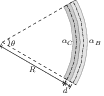# Thermal Expansion of Solids, Liquids, and Gases

## Problems from IIT JEE

Problem (IIT JEE 2008): An ideal gas is expanding such that $PT^2=\text{constant}$. The coefficient of volume expansion of the gas is,

1. $1/T$
2. $2/T$
3. $3/T$
4. $4/T$

Solution: The coefficient of volume expansion is defined as, \begin{align} \label{bga:eqn:1} & \gamma=\frac{1}{V}\frac{\mathrm{d}V}{\mathrm{d}T}. \end{align} The ideal gas equation, $PV=nRT$, gives $P=nRT/V$. Substitute $P$ in $PT^2=\text{constant}$ to get, \begin{align} \label{bga:eqn:2} & V=kT^3, \end{align} for some constant $k$. Differentiate second equation with respect to $T$ and substitute in first equation to get $\gamma=3/T$.

Problem (IIT JEE 2004): A cube of coefficient of linear expansion $\alpha_s$ is floating in a bath containing a liquid of coefficient of volume expansion $\gamma_l$. When the temperature is raised by $\Delta T$, the depth up to which the cube is submerged in the liquid remains the same. Find the relation between $\alpha_s$ and $\gamma_l$ showing all the steps.

Solution: Let cube has material density $\rho_s$, edge length $a$ and its height $h$ be inside the liquid of density $\rho_l$. The forces acting on the cube are its weight $a^3\rho_s g$ and the buoyancy force $a^2h\rho_l g$. In floating condition, \begin{align} \label{tsa:eqn:1} &a^3\rho_s g=a^2 h\rho_l g. \end{align} When temperature is increased by $\Delta T$, edge length of the cube increases to $a^\prime$ and density of the liquid decreases to $\rho_l^\prime$, given by, \begin{align} \label{tsa:eqn:2} a^\prime&=a(1+\alpha_s\Delta T),\\ \label{tsa:eqn:3} \rho_l^\prime&={\rho_l}/{(1+\gamma_l\Delta T)}. \end{align} Now, the volume of displaced liquid is $a^{\prime\,2}h$ and buoyancy force is $a^{\prime\,2}h\rho_l^\prime g$. Equate weight of the cube to the buoyancy force to get, \begin{align} \label{tsa:eqn:4} a^3\rho_s g&=a^{\prime\,2}hg \rho_l^\prime =a^2(1+\alpha_s\Delta T)^2 hg\left[{\rho_l}/{(1+\gamma_l\Delta T)}\right], \end{align} where we used second and third equations to eliminate $a^\prime$ and $\rho_l^\prime$. Divide first equation by fourth equation to get, \begin{align} &1+\gamma_l\Delta T=(1+\alpha_s\Delta T)^2\approx 1+2\alpha_s \Delta T,&&i.e., &&\gamma_l=2\alpha_s. \nonumber \end{align}

Problem (IIT JEE 2003): Two rods, one of aluminium and the other made of steel, having initial lengths $l_1$ and $l_2$ are connected together to form a single rod of length $l_1+l_2$. The coefficients of linear expansion for aluminium and steel are $\alpha_a$ and $\alpha_s$, respectively. If the length of each rod increases by the same amount when their temperature are raised by $t\;\mathrm{{}^oC}$, then find the ratio $\frac{l_1}{l_1+l_2}$,

1. $\frac{\alpha_s}{\alpha_a}$
2. $\frac{\alpha_a}{\alpha_s}$
3. $\frac{\alpha_s}{\alpha_a+\alpha_s}$
4. $\frac{\alpha_a}{\alpha_a+\alpha_s}$

Solution: After thermal expansion, length of the aluminium and the steel rod become, \begin{align} & l_1^\prime=l_1(1+\alpha_at),\\ & l_2^\prime=l_2(1+\alpha_st). \end{align} Since length of each rod is increased by the same amount, we get, \begin{align} &l_1^\prime-l_1=l_2^\prime-l_2. \end{align} Substitute $l_1^\prime$ and $l_2^\prime$ from first and second equation into third equation to get, $l_1\alpha_a=l_2\alpha_s$. Hence, \begin{align} \frac{l_1}{l_1+l_2}=\frac{l_1}{l_1+(l_1\alpha_a/\alpha_s)}=\frac{\alpha_s}{\alpha_a+\alpha_s}. \nonumber \end{align}

Problem (IIT JEE 1999): A bimetallic strip is formed out of two identical strips - one of copper and the other of brass. The coefficients of linear expansion of the two metals are $\alpha_C$ and $\alpha_B$. On heating, the temperature of the strip goes up by $\Delta T$ and the strip bends to form an arc of radius of curvature $R$. Then, $R$ is,

1. proportional to $\Delta T$.
2. inversely proportional to $\Delta T$.
3. proportional to $|\alpha_B -\alpha_C|$.
4. inversely proportional to $|\alpha_B -\alpha_C|$.

Solution:Let $d$ and $l_0$ be width and initial length of the two strips. The length of the strips after heating becomes, \begin{align} l_C&=l_0(1+\alpha_C\Delta T), \nonumber\\ l_B&=l_0(1+\alpha_B\Delta T).\nonumber \end{align} The strips bend as shown in the figure. From figure, the length of the two strips are \begin{align} l_C&=R\theta, \nonumber\\ l_B&=(R+d)\theta. \nonumber \end{align} Divide to get, \begin{align} \label{jqa:eqn:1} \frac{R+d}{R}&=\frac{l_B}{l_C}=\frac{1+\alpha_B\Delta T}{1+\alpha_C\Delta T}=(1+\alpha_B\Delta T)(1+\alpha_C\Delta T)^{-1}\nonumber\\ &\approx (1+\alpha_B\Delta T)(1-\alpha_C\Delta T)\approx 1+(\alpha_B-\alpha_C)\Delta T, \end{align} where we neglected higher order terms. Simplify above equation to get, \begin{align} \label{jga:eqn:2} R=\frac{d}{(\alpha_B-\alpha_C)\Delta T}. \end{align} The bending shown in figure assume $\alpha_B > \alpha_C$ (which is generally the case for brass and copper). If $\alpha_B < \alpha_C$, bending of strip will be in opposite direction. To take care of both cases, above equation is written as, \begin{align} R=\frac{d}{|\alpha_B-\alpha_C|\,\Delta T}.\nonumber \end{align}